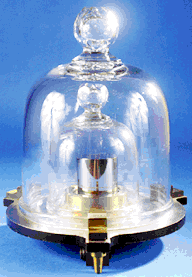# Measurement: Mass (Weight)

## The standard unit for measuring mass is the kilogram, kg. The kilogram is part of the metric system or ' The International System of Units (SI)' and was initially derived from the mass of a cubic decimeter or litre of water.

### These cylinders are called the'International Prototype Kilogram, IPK' and are kept at various secure locations around the world. They are held at atmospheric pressure in specially designed triple bell jars.### Mass is the amount of matter that an object contains and is measured in kilograms. The weight of an object is the force that is acting on the object due to gravity. Imagine an object with a mass of 1 kg. On Earth this object is pulled down by gravity and you feel and measure this force as weight. In space however there is no effect of gravity and the object would be weightless - but it would still have a mass of 1 kg. On the Moon, where the effect of gravity is 1/6th that on Earth, the same object would still have a mass of 1 kg but it would weigh 1/6th of what it weighs on Earth.

(Weight should technically not be measured in kilograms, The correct unit is the Newton, N, which is the force that the mass exerts due to gravity. 1 kg exerts a force of 9.8 Newtons.
So if your mass is 70 kg - you actually weigh 686 Newtons! This becomes more important when your child passes the 11 Plus, goes to grammar school and begins to study physics.)